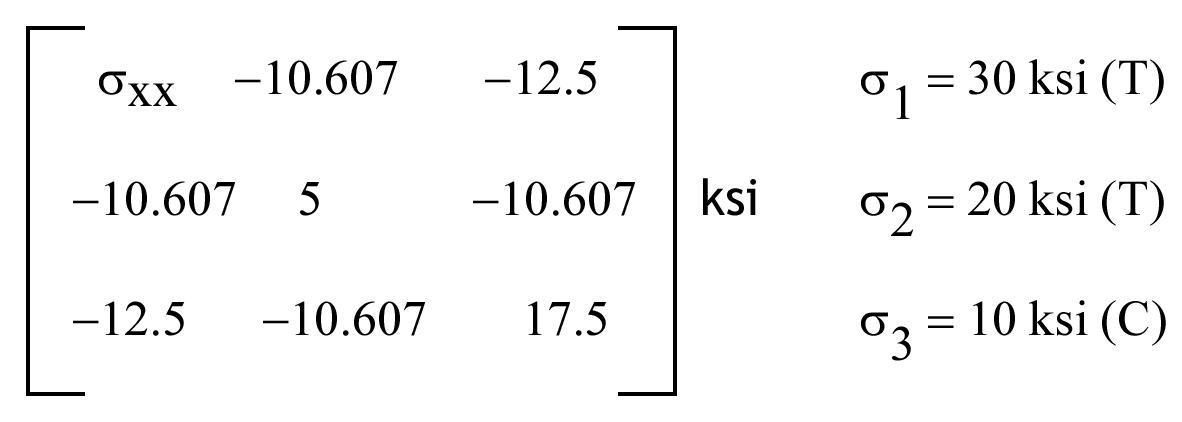# 1.3 – STRESS TRANSFORMATION

For each of the questions below, choose the best answer. The stress matrix and associated principal stresses are as given. Using this information choose the closest correct answer to 3 significant figures.Name Email
1. The first stress invariant is
2. The second stress invariant is
3. The third stress invariant is
4. The stress σxx is
5. The maximum shear stress is
6. The octahedral normal stress is
7. The magnitude of octahedral shear stress is
8. The magnitude of the stress vector on the plane whose outward normal makes the angle of 90o, 45o, 45o to the principal coordinates is
9. The magnitude of normal stress on the plane whose outward normal makes the angle of 90o, 45o, 45o to the principal coordinates is
10. The magnitude of shear stress on the plane whose outward normal makes an angle of 90o, 45o, 45o to the principal coordinates is